# Student's t-distribution facts for kids

Kids Encyclopedia Facts
Parameters Probability density function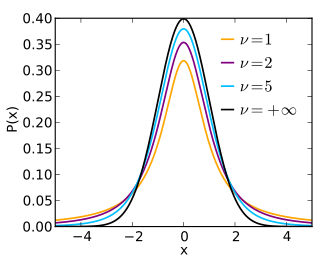Cumulative distribution function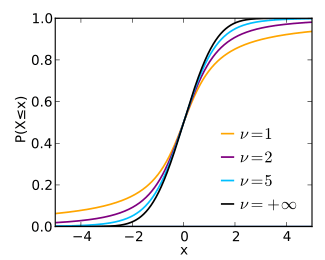ν > 0 degrees of freedom (real) x ∈ (−∞; +∞)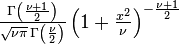$\textstyle\frac{\Gamma \left(\frac{\nu+1}{2} \right)} {\sqrt{\nu\pi}\,\Gamma \left(\frac{\nu}{2} \right)} \left(1+\frac{x^2}{\nu} \right)^{-\frac{\nu+1}{2}}\!$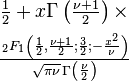$\begin{matrix} \frac{1}{2} + x \Gamma \left( \frac{\nu+1}{2} \right) \times\\[0.5em] \frac{\,_2F_1 \left ( \frac{1}{2},\frac{\nu+1}{2};\frac{3}{2}; -\frac{x^2}{\nu} \right)} {\sqrt{\pi\nu}\,\Gamma \left(\frac{\nu}{2}\right)} \end{matrix}$ where 2F1 is the hypergeometric function 0 for ν > 1, otherwise undefined 0 0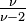$\textstyle\frac{\nu}{\nu-2}$ for ν > 2, ∞ for 1 < ν ≤ 2, otherwise undefined 0 for ν > 3, otherwise undefined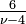$\textstyle\frac{6}{\nu-4}$ for ν > 4, ∞ for 2 < ν ≤ 4, otherwise undefined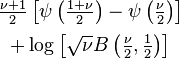$\begin{matrix} \frac{\nu+1}{2}\left[ \psi \left(\frac{1+\nu}{2} \right) - \psi \left(\frac{\nu}{2} \right) \right] \\[0.5em] + \log{\left[\sqrt{\nu}B \left(\frac{\nu}{2},\frac{1}{2} \right)\right]} \end{matrix}$ψ: digamma function, B: beta function undefined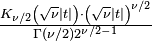$\textstyle\frac{K_{\nu/2} \left(\sqrt{\nu}|t|\right) \cdot \left(\sqrt{\nu}|t| \right)^{\nu/2}} {\Gamma(\nu/2)2^{\nu/2-1}}$ for ν > 0 Kν(x): Modified Bessel function of the second kind

Student's t-distribution is a probability distribution which was developed by William Sealy Gosset in 1908. Student is the pseudonym he used when he published the paper describing the distribution.

A normal distribution describes a full population, t-distributions describe samples drawn from a full population; accordingly, the t-distribution for each sample size is different, and the larger the sample, the more the distribution resembles a normal distribution.

The t-distribution plays a role in many widely used statistical analyses, including the Student's t-test for assessing the statistical significance of the difference between two sample means, the construction of confidence intervals for the difference between two population means, and in linear regression analysis. The Student's t-distribution also arises in the Bayesian analysis of data from a normal family.

## History

Gosset worked at a brewery and was interested in the problems of small samples, for example the chemical properties of barley. In the problems he analyzed, the sample size might be as low as three. Because of the small sample size, estimating the standard deviation is not possible. Also, in many cases Gosset encountered, the probability distribution of the samples was not known.

One version of the origin of the pseudonym is that Gosset's employer preferred staff to use pen names (instead of their real name) when publishing scientific papers, so he used the name "Student" to hide his identity. Another version is that the brewery did not want their competitors to know that they were using the t-test to test the quality of raw material.

## Properties

If we take a sample of n observations from a normal distribution, then the t-distribution with ν = n−1 degrees of freedom can be defined as the distribution of the location of the sample mean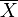$\overline{X}$, relative to the true mean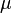$\mu$, divided by the sample standard deviation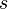$s$ over the normalizing term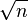$\sqrt{n}$ (that is,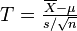$T = \tfrac{\overline{X}-\mu}{s/\sqrt{n}}$). In this way, the t-distribution can be used to estimate how likely it is that the true mean lies in any given range.

The t-distribution is symmetric and bell-shaped, like the normal distribution, but has heavier tails, meaning that it is more prone to producing values that fall far from its mean. This makes it useful for understanding the statistical behavior of certain types of ratios of random quantities, in which variation in the denominator is amplified and may produce outlying values when the denominator of the ratio falls close to zero. The Student's t-distribution is a special case of the generalised hyperbolic distribution.

## Related pages

• F-distribution

## Images for kidsStudent's t-distribution Facts for Kids. Kiddle Encyclopedia.